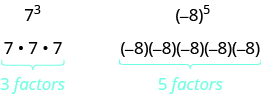## Simplifying Real Numbers With Exponents

### Learning Outcomes

• Simplify expressions with exponents and integer bases
• Simplify expressions with exponents and rational bases

Remember that an exponent indicates repeated multiplication of the same quantity. For example, ${2}^{4}$ means to multiply four factors of $2$, so ${2}^{4}$ means $2\cdot 2\cdot 2\cdot 2$. This format is known as exponential notation.

### Exponential NotationThis is read $a$ to the ${m}^{\mathrm{th}}$ power.

In the expression ${a}^{m}$, the exponent tells us how many times we use the base $a$ as a factor.Before we begin working with variable expressions containing exponents, let’s simplify a few expressions involving only numbers.

### example

Simplify:

1. ${5}^{3}$
2. ${9}^{1}$

Solution

 1. ${5}^{3}$ Multiply $3$ factors of $5$. $5\cdot 5\cdot 5$ Simplify. $125$
 2. ${9}^{1}$ Multiply $1$ factor of $9$. $9$

### example

Simplify:

1. ${\left({\Large\frac{7}{8}}\right)}^{2}$
2. ${\left(0.74\right)}^{2}$

### example

Simplify:

1. ${\left(-3\right)}^{4}$
2. ${-3}^{4}$